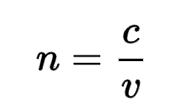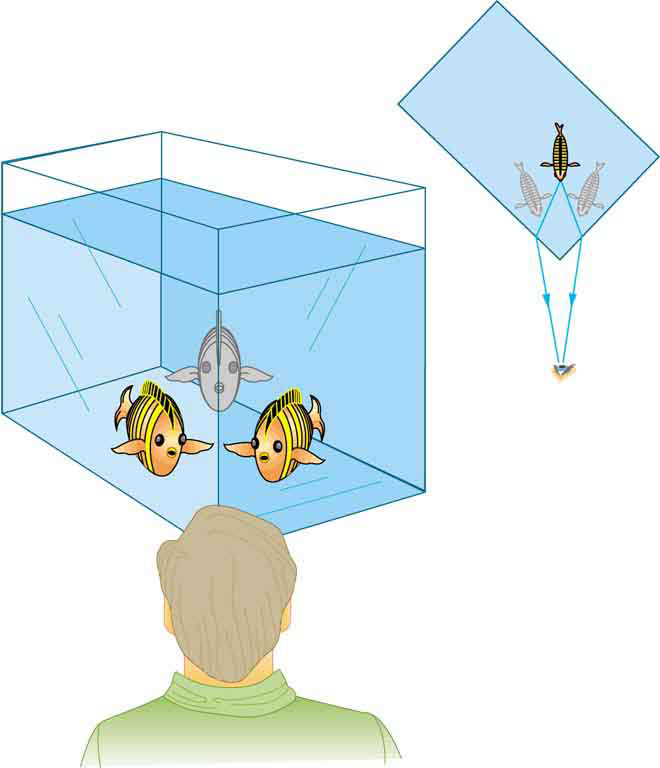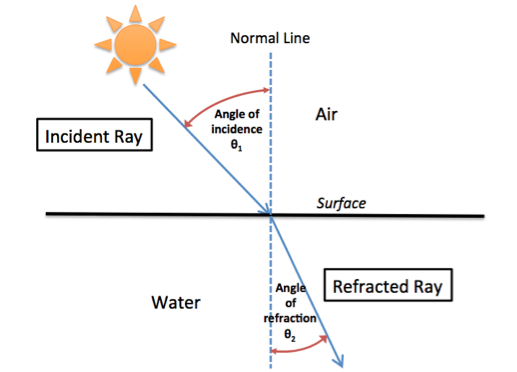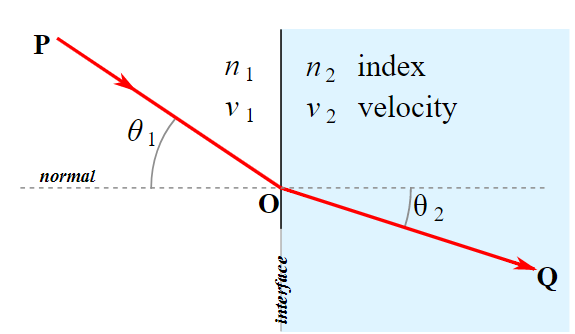# Bending Light Study Guide

INTRODUCTION

The most fundamental property we learn about light is that it travels straight. Yet, all-optical devices invented by humankind require light to bend. Lenses focus light to produce an image, optical fibers bend light in loops all-around your neighborhood to enable lightning-fast internet speed, and even the human eye bends light to create vision. While it is true that light’s direction can be changed by optical phenomena such as refraction and reflection, the path of light will always remain straight! So what really is this “bending of light”? Let’s find out!Source

### WHAT IS BENDING OF LIGHT?

The second fundamental property of light you would have heard is that it always travels at the same speed. Well, it is true only for a medium such as a vacuum. Whenever light travels through other optical mediums such as air, water, glass, diamonds, etc., it slows down!

Every time light slows down, something interesting happens- it changes direction. Multiple optical mediums in contact with one another can create interesting bending effects, a phenomenon called Refraction. To quantify how much the light slows down in any given medium, a dimensionless number called the refractive index is used.

The refractive index is the ratio of the speed of light in a vacuum to its speed in the given medium.

Mathematically it is represented as:Where n is the refractive index of the mediumc is the speed of lightv is the velocity of light in a given medium.

Mediums with a high refractive index bend light the most. The refractive index of the air is 1.0003 while water is 1.33. Diamonds have a refractive index of 2.4!

### REFRACTION OF LIGHTSource

The refraction of light is the reason why you see the fish in your aquarium at two different positions when you look at the tank from the side. Light bends and takes two different paths creating the effect. The bending of light through refraction is explained by Snell’s Law.Source

Before jumping into Snell’s law, we need to understand that whenever light travels from a rarer medium to a denser medium, it bends towards the normal line at the point of incidence (refer to figure above). The opposite is true when light travels from a denser medium to a rarer medium. Snell’s law gives the relationship between how much the light bends and the refractive index of the two mediums.

### SNELL’S LAW

According to Snell’s Law, for a given wavelength of light and pair of media, the ratio of the sine of the angle of incidence to the sine of the angle of refraction is a constant.Source

Mathematically it is expressed as:

``                      Sinθ1/Sinθ2 = n2/n1``

Or, n1 Sinθ1 = n2 Sinθ1

n1 is the refractive index of medium 1

n2 is the refractive index of medium 2

## CONCLUSION

• Whenever light travels from one medium to another, the bending of light is called refraction.
• The refractive index is the ratio of the speed of light in a vacuum to the speed of light in the medium.
• Snell’s law gives the relationship between how much the light bends and the refractive index of a pair of medium.

### FAQs:

1. What is bending of light?

The bending of light as it goes through one medium to another is called refraction.

2. Is light bending possible?

Yes, it is possible to bend light by changing the refractive index of the medium it is traveling in.

3. What materials can bend light?

Optical mediums such as glass, water, air of different densities, diamonds, and several other minerals such as ruby can bend light.

4. Can you bend light with a magnet?

Magnetic fields do not affect the photons of light.

5. What material bends light the most?

Materials with a high refractive index bend light the most, such as diamond (2.4).

We hope you enjoyed studying this lesson and learned something cool about Bending Light! Join our Discord community to get any questions you may have answered and to engage with other students just like you! We promise, it makes studying much more fun! 😎

## SOURCES:

1. 1.6 Refraction. https://flexbooks.ck12.org/cbook/cbse-physics-class-10/section/1.6/primary/lesson/refraction/. Accessed 10 Feb 2022.
2. Snells Law Formula. https://byjus.com/snells-law-formula/. Accessed 10 Feb 2022.
3. Snell’s Law. https://eng.libretexts.org/Bookshelves/Materials_Science/Supplemental_Modules_(Materials_Science)/Optical_Properties/Snell’s_Law. Accessed 10 Feb 2022.
4. Snell’s Law. https://www.physicsclassroom.com/class/refrn/Lesson-2/Snell-s-Law. Accessed 10 Feb 2022.
5. Refractive Index. https://byjus.com/physics/refractive-index/. Accessed 10 Feb 2022.
]]>